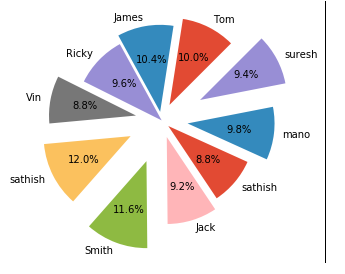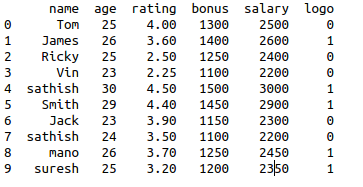• #5, First Floor, 4th Street Dr. Subbarayan Nagar Kodambakkam, Chennai-600 024 Landmark : Samiyar Madam
• pro@slogix.in
• +91- 81240 01111

### How to plot explode pie chart using matplotlib package in python?

###### Description

To plot explode pie chart for a data sample using python.

###### Process

Read the sample data.

Store it in a data frame.

Declare the values of explode.

Define the plotting variables.

Use plt.pie() to plot explode pie chart.

###### Sample Code

#import libraries

import matplotlib.pyplot as plt

import pandas as pd

#read the data set

/Sathish/Pythonfiles/Employee.csv’)

explode=(0.2,0.1,0.0,0.3,0.4,0.5,0.2,0.1,0.3,0.5)

#creating data frame

df=pd.DataFrame(data)

print(df)

#pie chart method and arguments

plt.pie(df[‘salary’],labels=df[‘name’],

explode=explode,startangle=

45,autopct=’%.1f%%’)

plt.show()

###### Screenshots